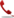+91-9818369374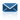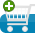Ask an Expert for Free Submit Your Assignment/Home work1 Live Tutoring Free Trial / 30 Min Sample Assignment / Home Work Make Payment Course Offered Resources
Quick Contact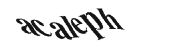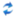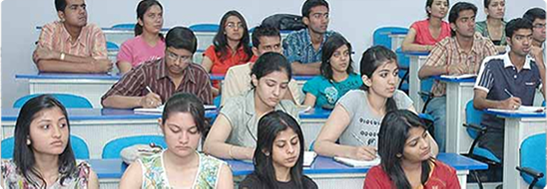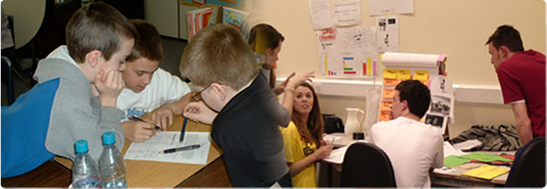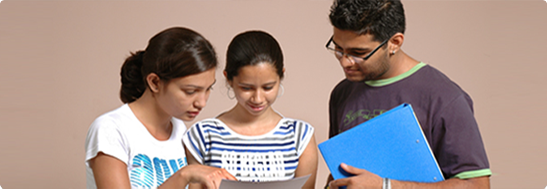# Question

To study the changes in velocity of a body pulled by a constant force

2. To study conservation of energy of a simple pendulum using ticker-timer and tape.

3. To study conservation of energy of a body falling freely using ticker and tape.

4. To study acceleration due to gravity with the help of ball rolling down an inclined plane and by applying correction for rotatory motion.

5. To study the acceleration of freely falling body using a ticker-timer and tape.

6. To study first law of motion by Galileo experiment using the double inclined track ie. a pair of inclined tracks each of which can be independently set at a small angle in inclination by plotting a graph between its sine (ie. perpendicular/hypotenuse) and the distance ascent.

7. To estimate the size of a molecule of an oil.

8. To study comparative cleansing effect of detergents through the study of surface tension.

9. To study the factors upon which the time period of simple pendulum depends.

10. To study the factors, affecting the force of friction (Static and Kinetic) between two surfaces.

11. To study the factors affecting the rate of loss of heat of a liquid.

12. To study the effect of surface on emission and absorption of radiation.

13. To study the factors affecting the refractive index of a material.

14. To study the factors affecting the resonant length of closed pipes.

15. To study a thermocouple and the variation of its thermo emf with temperature.

16. The study of the variations in intensity of a source of light with distance.

17. To study the motion of a ball using double inclined tracks/ channel.

18.To study various factors of dependence of internal resistance/ emf/ powers of different cells.

19. Investigate different factors of dependence of electric resistance by studying different kinds of materials (conductors, semiconductors, insulators).

20. To study the effectiveness of different materials for insulation of heat.

21. To study the various factors affecting the focal length of liquid lens.

22. To study the projective motion of a liquid jet under various conditions.

23.To study the effectiveness of different detergents in terms of quality and quantity

24. To study the infra-red radiations emitted by different sources using phototransistor.

25.To investigate the effect on the force of attraction between a solenoid and bar magnet when the current through the solenoid is changed.

26. To study the luminosity of various electric lamps of different makes and different powers.

27. To fabricate/ assemble fracting astronomical telescope and study its resolution with different apertures of object lens.

28. Study the variation of viscosity of solutions with concentration for different solutes using capillary flow.

29. Viscosities of viscous liquids using Stokes’ Method (Effect of addition of other liquids).

30. Study of absorption spectra using spectrometer.

31. Study of Emission Spectra using spectrometer.

32. Dependence of volume of liquid flowing through a capillary tube-on length & diameter of the tube and external pressure.

33. Verification

(1) Archimedes’ Principle

(2) Laws of floatation Applications

34. Parallax – Effect of parallax errors on measured length of a rod; reading in burette etc.

These are only specimens of suggestive Investigatory Projects. In order to promote innovativeness, the students be encouraged to take up new projects (other than the ones mentioned above) in consultation with and the approval of the teacher concerned.

Related posts:

To study the changes in velocity of a body pulled by a constant force

2. To study conservation of energy of a simple pendulum using ticker-timer and tape.

3. To study conservation of energy of a body falling freely using ticker and tape.

4. To study acceleration due to gravity with the help of ball rolling down an inclined plane and by applying correction for rotatory motion.

5. To study the acceleration of freely falling body using a ticker-timer and tape.

6. To study first law of motion by Galileo experiment using the double inclined track ie. a pair of inclined tracks each of which can be independently set at a small angle in inclination by plotting a graph between its sine (ie. perpendicular/hypotenuse) and the distance ascent.

7. To estimate the size of a molecule of an oil.

8. To study comparative cleansing effect of detergents through the study of surface tension.

9. To study the factors upon which the time period of simple pendulum depends.

10. To study the factors, affecting the force of friction (Static and Kinetic) between two surfaces.

11. To study the factors affecting the rate of loss of heat of a liquid.

12. To study the effect of surface on emission and absorption of radiation.

13. To study the factors affecting the refractive index of a material.

14. To study the factors affecting the resonant length of closed pipes.

15. To study a thermocouple and the variation of its thermo emf with temperature.

16. The study of the variations in intensity of a source of light with distance.

17. To study the motion of a ball using double inclined tracks/ channel.

18.To study various factors of dependence of internal resistance/ emf/ powers of different cells.

19. Investigate different factors of dependence of electric resistance by studying different kinds of materials (conductors, semiconductors, insulators).

20. To study the effectiveness of different materials for insulation of heat.

21. To study the various factors affecting the focal length of liquid lens.

22. To study the projective motion of a liquid jet under various conditions.

23.To study the effectiveness of different detergents in terms of quality and quantity

24. To study the infra-red radiations emitted by different sources using phototransistor.

25.To investigate the effect on the force of attraction between a solenoid and bar magnet when the current through the solenoid is changed.

26. To study the luminosity of various electric lamps of different makes and different powers.

27. To fabricate/ assemble fracting astronomical telescope and study its resolution with different apertures of object lens.

28. Study the variation of viscosity of solutions with concentration for different solutes using capillary flow.

29. Viscosities of viscous liquids using Stokes’ Method (Effect of addition of other liquids).

30. Study of absorption spectra using spectrometer.

31. Study of Emission Spectra using spectrometer.

32. Dependence of volume of liquid flowing through a capillary tube-on length & diameter of the tube and external pressure.

33. Verification

(1) Archimedes’ Principle

(2) Laws of floatation Applications

34. Parallax – Effect of parallax errors on measured length of a rod; reading in burette etc.

These are only specimens of suggestive Investigatory Projects. In order to promote innovativeness, the students be encouraged to take up new projects (other than the ones mentioned above) in consultation with and the approval of the teacher concerned.

Related posts:

1.    To study the changes in velocity of a body pulled by  a

constant force,

2.    To  study  the  conservation  of  energy  of  a  simple

pendulum using ticker-timer and a tape.

3.    To  study the conservation of energy of a body  falling

freely, using ticker-timer and tape.

4.    To study the acceleration due to gravity with the  help

of  ball rolling down an inclined plane and by  applying  the

correction for rotatory motion.

5.    To study the acceleration of freely falling body  using

a ticker-timer and a tape.

6.    To study the first law of motion by Galileo  experiment

using  the  double  inclined track i.e. a  pair  of  inclined

tracks  each  of which can be independently set  at  a  small

angle  in inclination by plotting a graph between  it's  sine

(i.e. perpendicular/hypotenuse) and the distance of ascent.

7.    To estimate the size of a molecule of an oil.

8.    To  study  the  comparative  cleansing  effect  of  the

different detergents through the study of surface tension.

9.    To  study the factors upon which the time period  of  a

simple pendulum depends.

54

10.   To  study the factors affecting the force  of  friction

(static and kinetic) between two surfaces.

11.   To study the factors affecting the rate of loss of heat

(or rate of cooling) of a liquid.

12.   To  study  the  effect  of  surface  on  emission   and

13.   To study the factors affecting the refractive index  of

a substance.

14.   To study the factors affecting the resonant lengths  of

closed pipes.

15.   To study thermocouple and the variation of its  thermo-

emf with temperature.

16.   To study the variations in the intensity of a source of

light with distance.

17.   To study the motion of a ball using the double inclined

track/channel.

Note: These are only specimens of Investigatory Projects.  In

order  to promote innovativeness, the students be  encouraged

to  take  up  new projects (other  than  the  ones  mentioned

above), in consultation with and the approval of the  teacher

concerned.   Many of the experiments demonstrated to  support

theory teaching can be extended for project work.

AssignmentWork Payment (USD)
Content